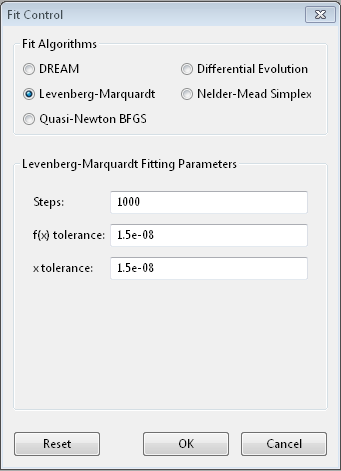# Optimizer Selection¶

Bumps has a number of different optimizers available, each with its own control parameters:

In general there is a trade-off between convergence rate and robustness, with the fastest algorithms most likely to find a local minimum rather than a global minimum. The gradient descent algorithms (Levenberg-Marquardt, Quasi-Newton BFGS) tend to be fast but they will find local minima only, while the population algorithms (DREAM, Differential Evolution) are more robust and likely slower. Nelder-Mead Simplex is somewhere between, with a small population keeping the search local but more robust than the gradient descent algorithms.

Each algorithm has its own set of control parameters for adjusting the search process and the stopping conditions. The same option may mean slightly different things to different optimizers. The Bumps package provides a dialog box for selecting the optimizer and its options when running the fit wx application. This only includes the common options for the most useful optimizers. For full control, the fit will need to be run from the command line interface or through a python script.

For parameter uncertainty, most algorithms use the covariance matrix at the optimum to estimate an uncertainty ellipse. This is okay for a preliminary analysis, but only works reliably for weakly correlated parameters. For full uncertainty analysis, DREAM uses a random walk to explore the parameter space near the minimum, showing pair-wise correlations amongst the parameter values. In order for DREAM to return the correct uncertainty, the function to be optimized should be a conditional probability density, with nllf as the negative log likelihood function of seeing point $$x$$ in the parameter space. Other functions can be fitted, but uncertainty estimates will be meaningless.

Most algorithms have been adapted to run in parallel at least to some degree. The implementation is not heavily tuned, either in terms of minimizing the overhead per function evaluation or for distributing the problem across multiple processors. If the theory function is implemented in parallel, then the optimizer should be run in serial. Mixed mode is also possible when running on a cluster with a multi-threaded theory function. In this case, only one theory function will be evaluated on each cluster node, but the optimizer will distribute the parameters values to the cluster nodes in parallel. Do not run serial algorithms (Levenberg-Marquardt, Quasi-Newton BFGS) on a cluster.

We have included a number of optimizers in Bumps that did not perform particularly well on our problem sets. However, they may be perfect for your problem, so we have left them in the package for you to explore. They are not available in the GUI selection.

## Levenberg-Marquardt¶The Levenberg-Marquardt   algorithm has been the standard method for non-linear data fitting. As a gradient descent trust region method, it starts at the initial value of the function and steps in the direction of the derivative until it reaches the minimum. Set up as an explicit minimization of the sum of square differences between theory and model, it uses a numerical approximation of the Jacobian matrix to set the step direction and an adaptive algorithm to set the size of the trust region.

### When to use¶

Use this method when you have a reasonable fit near the minimum, and you want to get the best possible value. This can then be used as the starting point for uncertainty analysis using DREAM. This method requires that the problem definition includes a residuals method, but this should always be true when fitting data.

When modeling the results of an experiment, the best fit value is an accident of the measurement. Redo the same measurement, and the slightly different values you measure will lead to a different best fit. The important quantity to report is the credible interval covering 68% (1-$$\sigma$$) or 95% (2-$$\sigma$$) of the range of parameter values that are somewhat consistent with the data.

This method uses lmfit from scipy, and does not run in parallel.

### Options¶

Steps is the number of gradient steps to take. Each step requires a calculation of the Jacobian matrix to determine the direction. This needs $$2 m n$$ function evaluations, where $$n$$ is the number of parameters and each function is evaluated and $$m$$ data points (assuming center point formula for finite difference estimate of the derivative). The resulting linear equation is then solved, but for small $$n$$ and expensive function evaluation this overhead can be ignored. Use --steps=n from the command line.

f(x) tolerance and x tolerance are used to determine when the fit has reached the point where no significant improvement is expected. If the function value does not improve significantly within the step, or the step is too short, then the fit will terminate. Use --ftol=v and --xtol=v from the command line.

From the command line, --starts=n will automatically restart the algorithm after it has converged so that a slightly better value can be found. If --keep_best is included then restart will use a value near the minimum, otherwise it will restart the fit from a random point in the parameter space.

Use --fit=lm to select the Levenberg-Marquardt fitter from the command line.